## Speed Torque Characteristic of Separately Excited DC Motor:

The circuit equation of a Speed Torque Characteristic of Separately Excited DC Motor whose armature, having a total of Z con­ductors, is wound for 2P poles (the brushes divide the winding into 2a parallel paths), is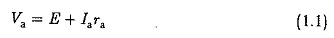Where E is the back emf of the armature given by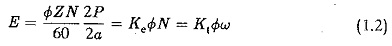where ω is the angular velocity given by ω = (2π N/60), Ke and Kt can be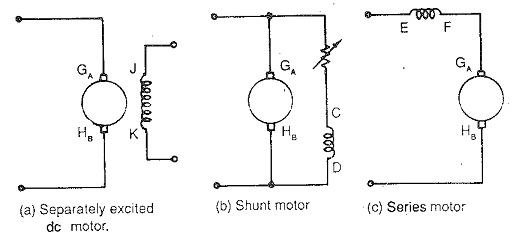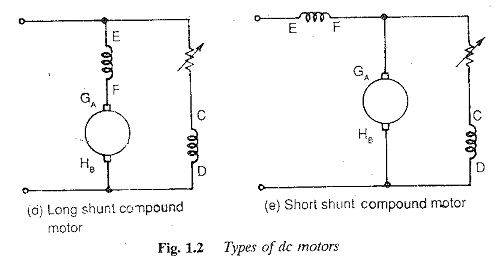From Eqs (1.1) and (1.2) we get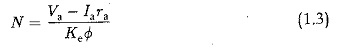The torque developed by the motor is given by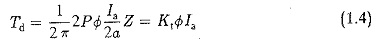Substituting for Ia in Eq. (1.3) from Eq. (1:4) we have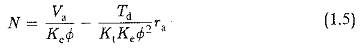When Td = 0 the corresponding speed N0=Va/(KeΦ) is the no-load speed. The motor speed decreases as the torque developed increases, resulting in a drooping characteristic. The speed-torque curves are shown in Fig. 1.3. The figure clearly shows a speed drop of 2 to 3% as the torque varies from no-load to full load.

In dc machines the armature mmf reacts with the field mmf this reaction is known as the armature reaction. When the effects of the armature reaction are neglected, the flux per pole of the motor is constant and is independent of load. In normal construction the brushes are placed in the neutral zone. The armature reaction, though cross magnetising, is followed by demagnetisation due to saturation. The effect of demagnetisation on the field flux due to armature flux is clearly shown in Fig. 1.3. The speed drop from no-load to full load decreases, improving speed regulation.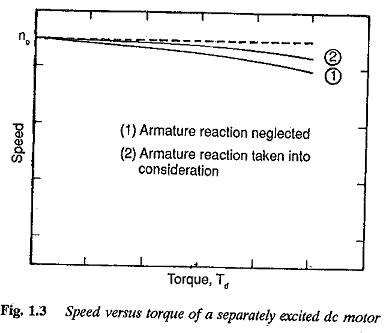The effect of additional resistance in the armature circuit is depicted in Fig. 1.4. Speeds in the range of zero to base speed may be obtained. With a suitable value of ra very slow speeds are possible, at the cost of efficiency.

The speed-torque curves for a smooth variation of armature voltage are shown in Fig. 1.5. They move along the Y-axis (speed axis) following changes in the armature voltage. The field winding of the motor is supplied from a separate source. The smooth variation of armature voltage brings about speed control in the zero to base speed range very efficiently. The motor operates in a constant torque mode. This method of controlling the speed of a Separately Excited DC Motor using variable voltage to the armature is employed in Ward Leonard control.

The effect of the variation of field current on the speed-torque characteristic is shown in Fig. 1.6. The field current is decreased to achieve speeds above base speed when the armature voltage reaches its rated value. The flux weakening mode is best suited for constant power applications, since the armature current may be maintained at its rated value. The torque decreases. In the flux weakening mode the motor cannot be used to drive constant torque loads as the motor draws increased currents as the speed increases. This mode is employed to obtain speeds in the range of base speed to twice base speed. The highest speed attainable by flux weakening is limited by commutation.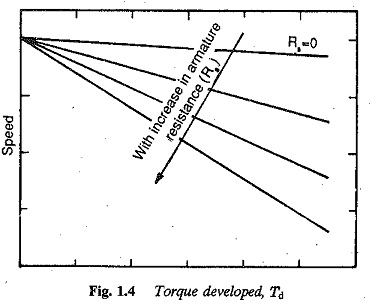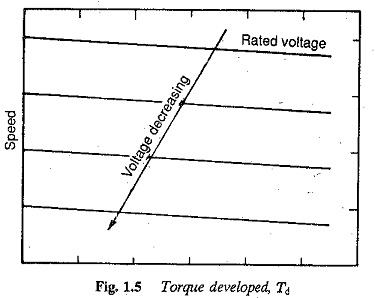The armature current can remain constant in the complete range of speeds from zero to twice base speed. Constant torque and constant power modes are shown in Fig. 1.7. The operation depicted in Fig. 1.7 is possible with shunt motors also by means of a variable resistance in the field circuit.